# Power Factor

Power factor is the measure of how effectively the incoming power is used in an electrical system. A high power factor indicates that the power supplied to the electrical system is effectively used. A system with low power factor doesn’t effectively consume the incoming electric supply and results in losses. There is no power factor involved in DC circuits due to zero frequency. But, in AC circuits, the value of power factor always lies between 0 and 1.

## Explaining Power Factor

Power factor is defined as the cosine of the angle between voltage and current. Ideally, in AC circuits, the phase difference between voltage and current is zero. But, practically there exists some phase difference between the two. The cosine of the phase difference between the two is defined as the power factor.

### Power Factor Calculation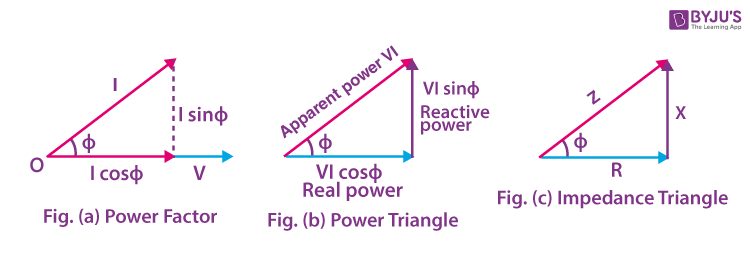In the figure, angle ɸ is the phase difference between the voltage and current phasor. Therefore, the power factor is:

Power Factor = cosɸ

In the figure of the power triangle,

VI sinɸ = Reactive power (in VAR)

VI cosɸ = Active power (in Watts)

VI = Apparent power (in VA)

PF = cosɸ = Active Power (W) / Apparent Power (VA)

 Knowledge Review Real Power – The power supplied to the equipment that performs useful and productive work. Reactive Power – The power required by equipment such as transformers and motors to produce magnetic fields enabling actual work to be done. Apparent Power – The vector sum of real power and reactive power

### Power Factor can be Lagging, Leading or Unity

#### Lagging Power Factor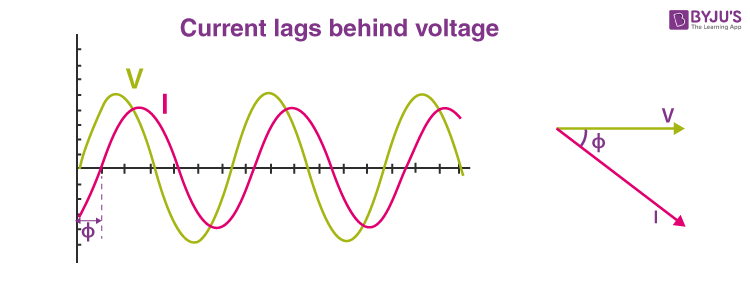In a circuit, when the current lags behind the voltage, then the power factor of the circuit is known as a lagging power factor. The power factor lags when the circuit is inductive. Loads such as coils, motors and lamps are inductive and have lagging pf.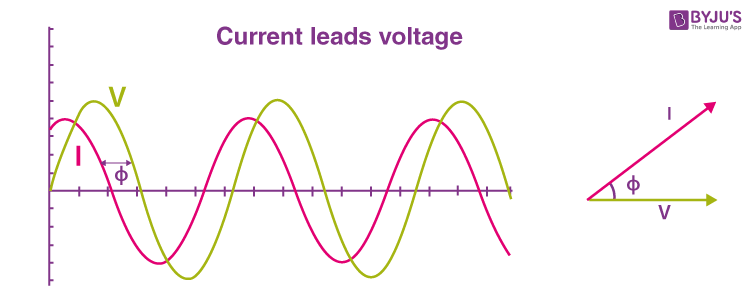When the current in the circuit leads the voltage, then the power factor of the circuit is said to be leading. A capacitive circuit has a leading power factor. Capacitor banks and Synchronous condensers are capacitive loads that have a leading power factor.

#### Unity Power Factor

The power factor is unity for ideal circuits. The power factor is unity when the current and voltage are in phase.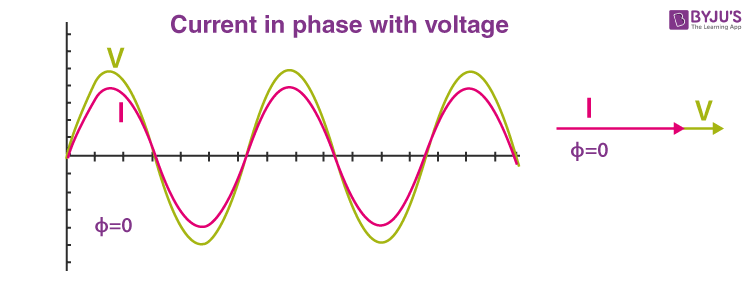## Effects of Low Power Factor

Here are some disadvantages of low power factor:

### Large Copper Losses

The copper losses are directly proportional to the square of the line current and the line current is inversely proportional to the power factor of the circuit. Combining these two relations, we understand that the copper losses will be inversely proportional to the square of power factor. When the power factor is low, the line current will be high, and consequently, the copper losses will be higher. This results in low efficiency of the power system.

### Large kVA rating

Electric machines such as transformers are rated in kVA. The relationship between the power factor and the kVA rating of the machine is given by the formula below:

kVA = kW/ CosФ

From the formula, we know that the power factor of the machine is inversely proportional to its kVA rating. Larger kVA rating makes the equipment costly and heavier in size.

### Poor Voltage Regulation

We already know that poor power factor results in a larger line current to be drawn by the electrical equipment. As a result, a large current at a low lagging power factor causes a higher voltage drop in transformers and alternators. This results in the decreased voltage available at the supply end of equipment and hence poor voltage regulation.

## Causes of Low Power Factor

In this section, we will discuss some of the main causes of low power factor.

### Harmonic Current

The presence of harmonic current reduces the power factor in the system.

### Improper Wiring

Due to improper wiring or electrical accidents, an imbalance in the 3- phase power occurs which causes low power factor.

The load demand in the modern power system is not constant throughout the day. The demand is high during the morning and evening hours and the light during the rest of the day. When the system is loaded lightly, the voltage increases, increasing the magnetization current demand of the machine. This causes a poor power factor in the system.

90% of the industrial loads consist of induction motors. Such machines draw magnetizing current and set up a magnetic field for its proper working and hence work at a low power factor.

The current drawn by inductive loads is lagging and results in poor power factor.

## Power Factor Correction

The power factor correction can be done by the following two methods:

• Power Factor Correction Method using Capacitors
• Power Factor Correction Method using a Synchronous Condenser

### Power Factor Correction by Capacitor Banks

In a three-phase system, the power factor is improved by connecting capacitors in star or delta. The star and delta connections of the capacitor banks are shown in the diagram below: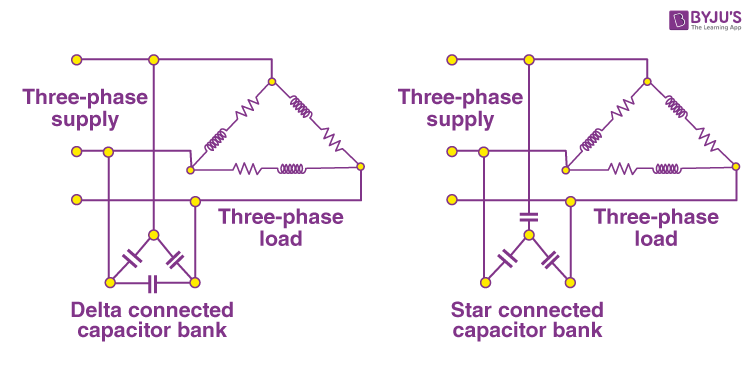Let,

VL be the Line voltage

Cy = capacitance per phase when the capacitor is connected in stars

CΔ = capacitor per phase when the capacitors are connected in delta

Qc = Var rating of each phase

Vp be the phase voltage

Delta Connection

For delta connection,

Vp = VL

The capacitance per phase is given by the equation:

$$\begin{array}{l}C=\frac{Q_{c}}{\omega V_{p}^2}=\frac{Q_{c}}{\omega V_{L}^2}\end{array}$$
……(eqn 1)

Star Connection

For delta connection,

$$\begin{array}{l}V_{P}=\frac{1}{3}V_{L}\end{array}$$

The capacitance per phase is expressed by the equation:

$$\begin{array}{l}C_{Y}=\frac{Q_{c}}{\omega V_{p}^2}=\frac{Q_{c}}{\omega {(\frac{V_{L}}{\sqrt{3}}})^2}=\frac{3Q_{c}}{\omega(V_{L})^2}\end{array}$$
……(eqn 2)

From equation (1) and (2) we get

$$\begin{array}{l}C_{Y}=3C_{\Delta}\end{array}$$

From equation 3, we infer the following:

• The capacitance needed in a star connected three-phase transformer is equal to three times the capacitance required per phase when the capacitors are connected in delta.
• The working voltage of the star connected bank is 1/√3 times the delta connected bank.

Due to the above observations, the capacitors are connected in the delta in a three-phase system for power factor improvement.

### Power Factor Correction by Synchronous Condenser

Synchronous condensers are specially designed induction motors. When connected in parallel to the load, the synchronous condenser absorbs and generates the reactive power (Var) by varying the excitation of the motor field winding. Synchronous Condenser, however, has some disadvantages such as it is costly and its installation and maintenance is not easy.

## Frequently Asked Questions on Power Factor

Q1

### What is the power factor?

Power factor is defined as the cosine of the angle between voltage and current. Power factor is the measure of how effectively the incoming power is used in the electrical system.

Q2

### How is the power factor measured?

The power factor is the cosine of the phase difference between voltage and current, and as a result, the power factor is measured using the formula Power Factor = cosɸ, where ɸ is the phase difference between the voltage and current phasor.

Q3

### What are the causes of low power factor?

Some causes of low power factor are a) The presence of harmonic current in the system reduces power factor. b) Improper wiring leads to three-phase imbalance causing low power factor. c) When the system is loaded lightly, the voltage increases, increasing the magnetization current demand of the machine. This causes a poor power factor in the system. d) The current drawn by inductive loads is lagging and results in a poor power factor.

Q4

### What are the effects of low power factor?

Some effects of low power factor are as follows: a) Large Copper Losses b) Large kVA rating c) Poor Voltage Regulation.

Q5

### What is the low power factor correction?

Low power factor correction is a method of improving the power factor and therefore, improving the power quality. The low power factor can be corrected using a) capacitor banks and, b) Synchronous Condenser.

Stay Tuned to BYJU’S and Fall in Love with Learning!